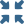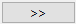# 创建结果封套

$\left\{{R}_{s}\right\}\text{\hspace{0.17em}}=\text{\hspace{0.17em}}\left(\text{min/max/extreme}\right)\text{\hspace{0.17em}}\left\{{c}_{1}\left\{{R}_{1}\right\},\text{\hspace{0.17em}}{c}_{2}\left\{{R}_{2}\right\},\text{\hspace{0.17em}}\dots \text{\hspace{0.17em}}{c}_{i}\left\{{R}_{i}\right\}\right\}$

${R}_{i}$

${c}_{i}$

1. 在主工具栏上，选择（后研究）
2. 选择结构封套
3. 从下拉菜单中选择封套分析类型。
4. 在对话框中，按住 控制 + Shift 并选择要合并的结果。
5. 点击按钮，合并分析。
6. 点击求解
结构封套支持结果图和拾取信息后处理选项。